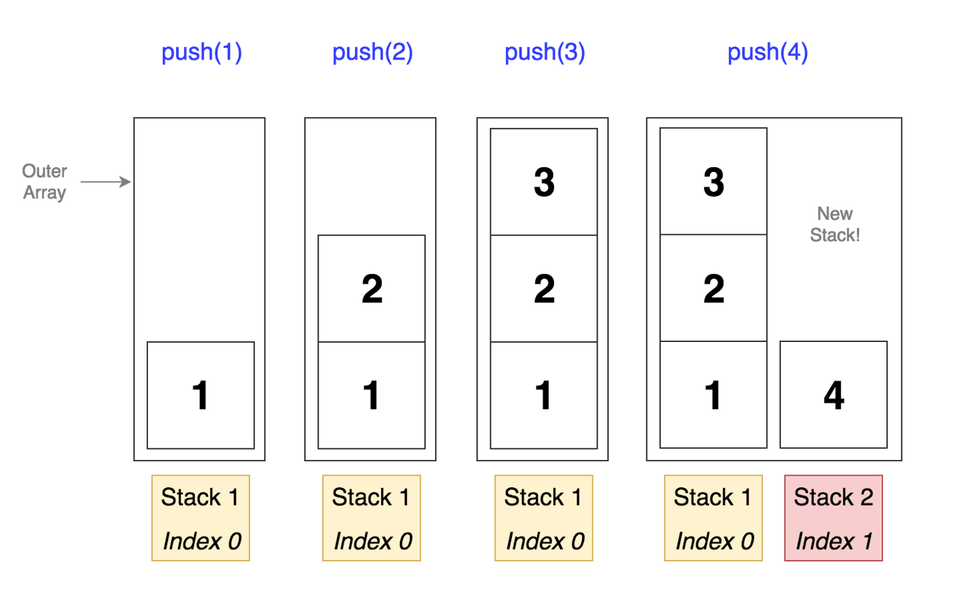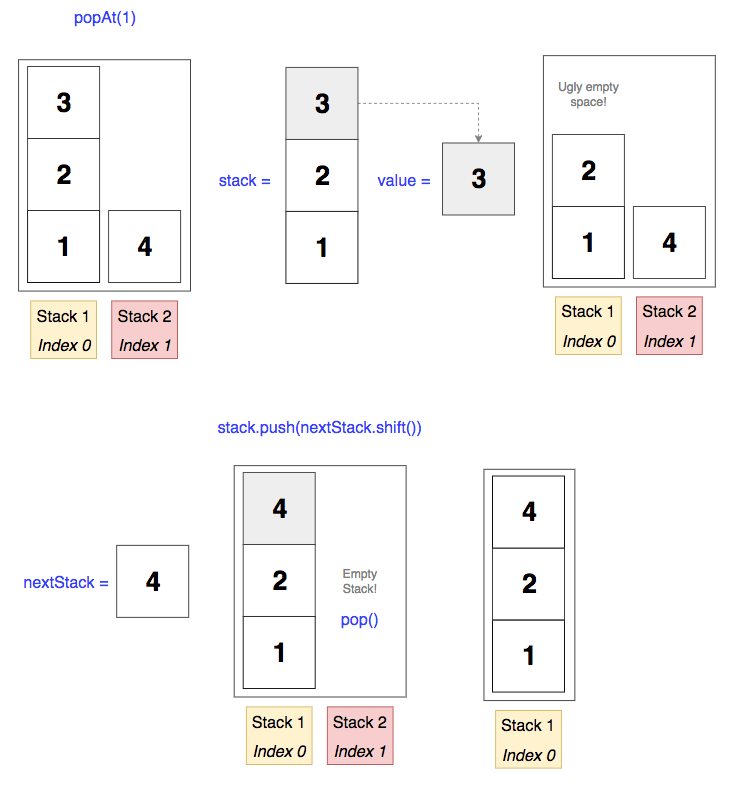How To: Implement a Set of Stacks in Javascript

The problem: create a stack data structure that will create additional stacks if the previous stack exceeds a particular size.

Pushing and popping from this stack should function as it normally would in a regular stack. That is, push() should place a value at the end of the last stack and pop() should return the last value in the last stack.

Bonus: create a popAt() method that returns a popped value from a particular stack and shifts the rest of the values so there are no gaps.

Plan of Action

So we know we’ll at least need to start things off with an empty master stack which we’ll eventually put more stacks into as we accumulate input. We’ll probably also want a variable where we can store the maximum stack size that a user can pass in.

Our push() method needs to find the last stack and push a value into it. It also needs to check if that stack exceeds the maximum size, because if it does, it will need to push another empty stack into our master stack.

Our pop() method similarly needs to find the last stack and pop the last value from it. It also needs to check the length of the last stack and if there’s nothing left, pop it from the master stack so we don’t have empty stacks lying around.

Our popAt() method will be the most complex. It will take a number that corresponds to the stack where we want to pop a value. First, we should check the number against our stack length because we know if the number is equal to the number of stacks, we can just run our regular pop method because it’s simply popping the last value from the last stack.

We should also check to see if the number is out of bounds in any way, like if it’s 0 or if it’s greater than the number of stacks we have. If so, then that would be an error.

Finally, we’ll need to find the stack in question, pop the value from that stack, then shift the rest of the stacks in a way that leaves no leftover space. The solution for this may not be immediately obvious, but we’ll see it’s actually simpler than you may think.

Step 1: Initialize Our Data structure

1class StackSet {
2 constructor(maxSize) {
3 if (arguments.length < 1) {
4 throw new Error (‘Woops, maxSize is required!)
5 }
6
7this.stacks = [[]]
8this.maxSize = maxSize
9}
10}

Since we know we’ll eventually have multiple stacks, we can start off with a nested array. This makes things a bit easier to get started.

Additionally, we need to store the maximum size since that’s what will determine when we create a new stack.

Step 2: Our push() Method

1push(value) {
2 if (this.stacks[this.stacks.length - 1].length === this.maxSize) {
3 this.stacks.push([])
4 }
5
6this.stacks[this.stacks.length - 1].push(value)
7}

First we create our push() method with a value param since we want to add a value to our stacks. Then we’ll do a quick check and see if the last stack in our master stack equals the max size passed into our constructor above. If so, we know we need another stack in our master stack because we can’t fit any more in the current stack, so let’s push an empty one.

Lastly, we’ll push our value into the last stack.

Note: remember that .length returns the actual length of an array, not the number of indices. That’s why we need to subtract 1.

Let’s say our maxSize = 3. Here’s a visualization of what’s happening:Step 3: Our popAt() Method

1popAt(number) {
2 // checks
3 if (number < 1 || number > this.stacks.length) {
4 throw new Error ('Whoa, that number is either too small or too large for our stack.')
5 }
6
7 if (number === this.stacks.length) {
8 return this.pop()
9 }
10
11 let stack = this.stacks[number - 1]
12 let value = stack.pop()
13 let nextStack = []
14
15 for (let i = number; i < this.stacks.length; i++) {
16 nextStack = this.stacks[i]
17 nextStack.reverse()
18 stack.push(nextStack.pop())
19 nextStack.reverse()
20 stack = nextStack
21 }
22
23 if (this.stacks.length > 1 && this.stacks[this.stacks.length - 1].length === 0) {
24 this.stacks.pop()
25 }
26
27 return value;
28}

Remember this method only wants one popped value from the stack at the number passed in. And we need to shift our stacks so there aren’t any spaces.

At the top we can start with some initial checks. If the number is 0 or larger than the number of stacks, then it’s of no use to us so we should let the user know.

Next, if the number is the same as the last stack, then we can run a regular pop(). No sweat.

If we don’t meet any of those initial checks, now it’s time to do work.

First, let’s grab the stack we need by subtracting 1 from the number param to get our stack index. Good, now let’s pop off the value we ultimately want to return from that stack.

We’re almost there, now we just need to clean up our stacks.

To do our shifting, we‘ll run a for loop on our stacks, starting at the stack that corresponds to our numbers param (we don’t care about the stacks that come before because they aren’t affected by the pop).

Basically what we want to do is 1) grab the stack after our selected stack, 2) pop off the first value of that stack and 3) add the popped value to our selected stack since there’s an empty spot there where we popped off the final value earlier. We can do this pretty painlessly with the shift() method which acts like pop(), but on the beginning of an array instead of the end.

Remember that arrays and objects in Javascript are assigned by reference meaning when you assign a variable to an array or object it points to that actual object instead of creating a copy. That means when we’re modifying ‘stack’ and ‘nextStack’, we’re modifying the stacks in our master stack.

After that, we’ll assign stack to whatever’s left over in nextStack, that way if our loop has any more iterations left, it can run the same process on the next stack until everything is in its right place.

Finally, we’ll run the same check we did in pop() to see if after all the work we did the last stack is empty. If so, let’s get rid of it. Then we’ll ultimately return our popped value so the user can see what it was.The Final Code

Here’s what our data structure will look like. I’ve added some logs at the end to show you what the output will be for each method. If you notice any typos, let me know!

1class StackSet {
2 constructor(maxSize) {
3 if (arguments.length < 1) {
4 throw new Error("Woops, maxSize is required!");
5 }
6
7 this.stacks = [[]];
8 this.maxSize = maxSize;
9 }
10
11 push(value) {
12 if (this.stacks[this.stacks.length - 1].length === this.maxSize) {
13 this.stacks.push([]);
14 }
15
16 this.stacks[this.stacks.length - 1].push(value);
17 }
18
19 pop() {
20 const value = this.stacks[this.stacks.length - 1].pop();
21
22 if (
23 this.stacks.length > 1 &&
24 this.stacks[this.stacks.length - 1].length === 0
25 ) {
26 this.stacks.pop();
27 }
28
29 return value;
30 }
31
32 popAt(number) {
33 if (number < 1 || number > this.stacks.length) {
34 throw new Error(
35 "Whoa, that number is either too small or too large for our stack."
36 );
37 }
38
39 if (number === this.stacks.length) {
40 return this.pop();
41 }
42
43 let stack = this.stacks[number - 1];
44 let value = stack.pop();
45 let nextStack = [];
46
47 for (let i = number; i < this.stacks.length; i++) {
48 nextStack = this.stacks[i];
49 stack.push(nextStack.shift());
50 stack = nextStack;
51 }
52
53 if (
54 this.stacks.length > 1 &&
55 this.stacks[this.stacks.length - 1].length === 0
56 ) {
57 this.stacks.pop();
58 }
59
60 return value;
61 }
62}
63// initialize new StackSet object
64const myStack = new StackSet(3);
65// to test if it works
66myStack.push(1);
67myStack.push(2);
68myStack.push(3);
69myStack.push(4);
70myStack.push(5);
71myStack.push(6);
72console.log(myStack.stacks); // [ [ 1, 2, 3 ], [ 4, 5, 6 ] ]
73console.log(myStack.pop()); // 6
74console.log(myStack.stacks); // [ [ 1, 2, 3 ], [ 4, 5 ] ]
75console.log(myStack.popAt(1)); // 3
76console.log(myStack.stacks); // [ [ 1, 2, 4 ], [ 5 ] ]
1